Get instant live expert help with Excel or Google Sheets“My Excelchat expert helped me in less than 20 minutes, saving me what would have been 5 hours of work!”

#### Post your problem and you’ll get Expert help in seconds.

Your message must be at least 40 characters
Our professional Expert are available now. Your privacy is guaranteed.

# Use Data Validation in Excel to Allow Text Only

We can use the ISTEXT function to allow only text in a cell. This data validation formula will make excel allow only the kind of data we want. In a few steps below, we will learn how to use this formula.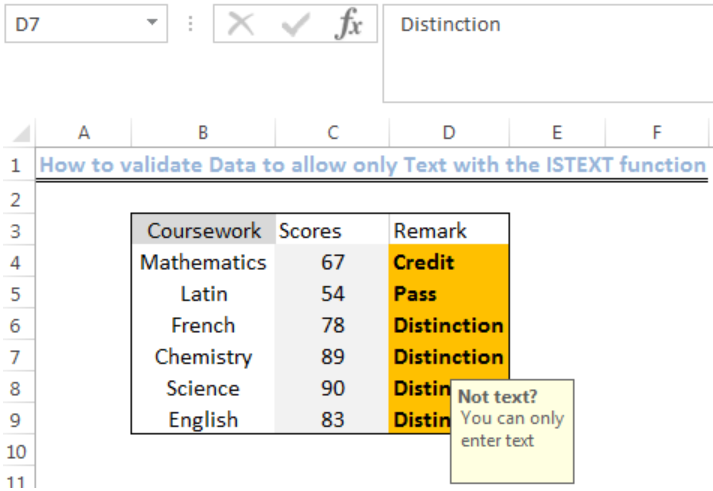Figure 1: Result of applying the ISTEXT function

## General Formula

`=ISTEXT(A1)`

## Formula

`=ISTEXT(D4)`

## Setting up the Data

• We will set up our data titled Coursework in Column B
• The Scores for each course will be entered in Column C
• Column D is where we will introduce the formula to return the result titled Remark.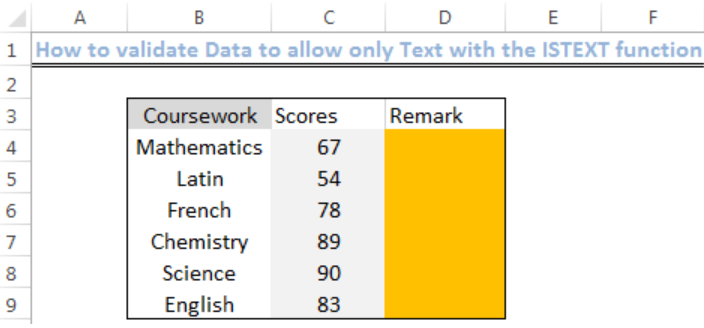Figure 2: Setting up the Data

## Validate Data to Allow Text Only

• We will highlight the range D4:D9 to be validated
• We will go to the Data Tab at the top of the excel sheet
• We will click on “Data Validation,”
• In the Allow field, we will select custom and insert the formula: `=ISTEXT(D4)`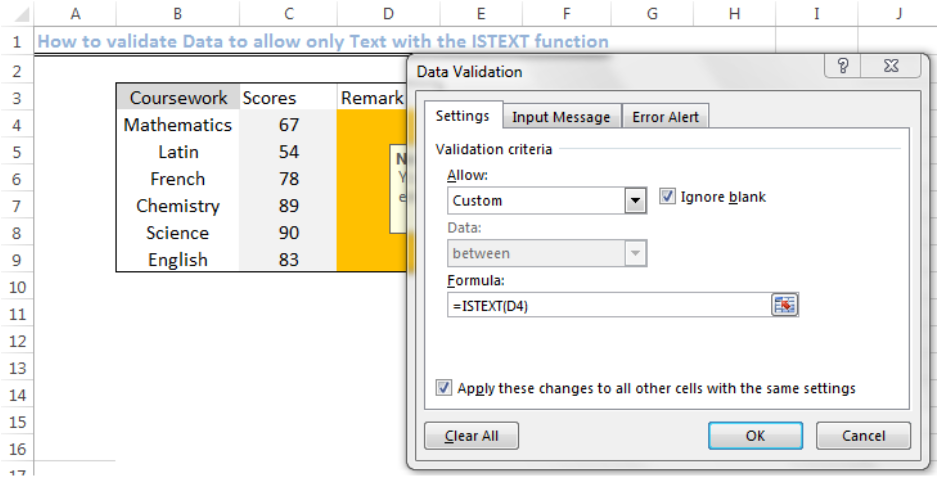Figure 3: Applying the Data Validation function

• In the Message tab, we will write the error title and error message we wish to see.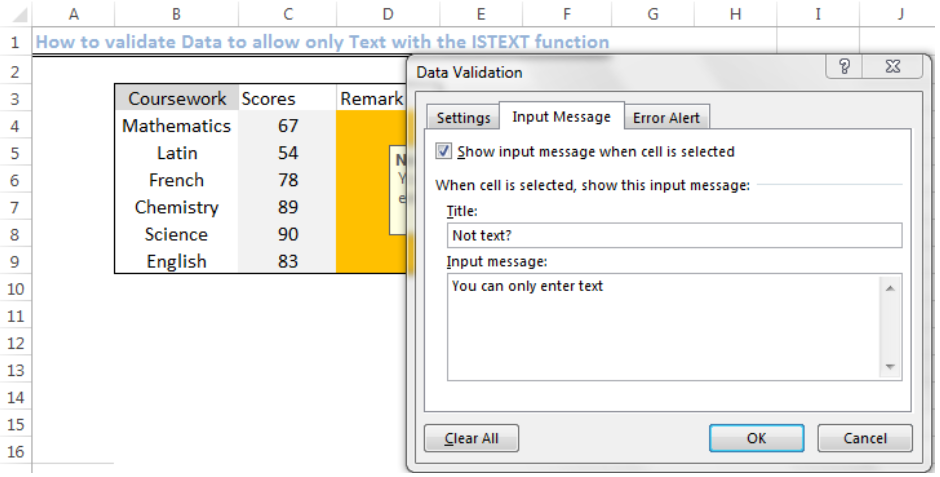Figure 4: Input the Error text message

• Now, we will click “OK” to apply
• We will click Cell D4 and insert the text we want to see (in this case, “Credit”)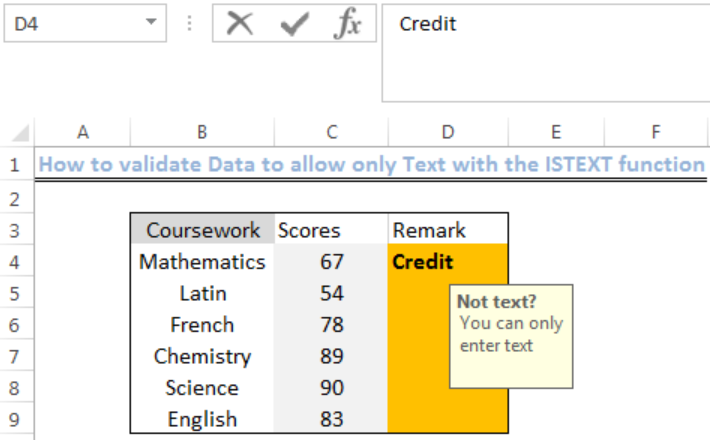Figure 5: If text, then return TRUE

• In Cell D5, we enter a value, and it returns with the error message.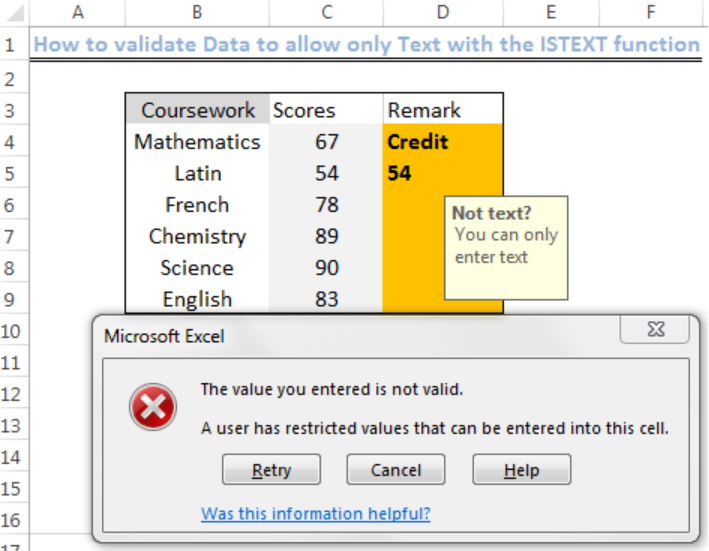Figure 6: Entered Value is not a Text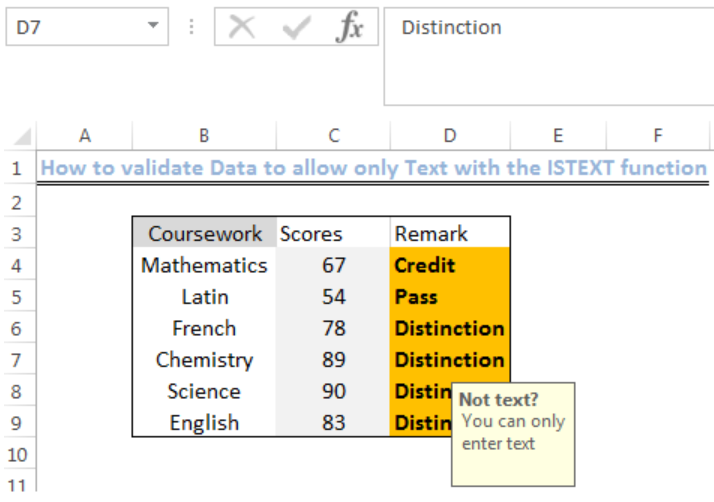Figure 7: Final Sheet with Text

## Explanation

`=ISTEXT(D4)`

We use the Data validation function and to restrict the kind of data we want in a cell when a user adds or wishes to change the cell value. The ISTEXT function returns TRUE if the value is text and FALSE if not. Because of this, a text will pass the validation, but numbers and formulas will not.

## Notes

• We should ensure that the “ignore blank” is clicked
• We  can apply the Data validation window minus the input message
• There are many other Data validation functions apart from ISTEXT function
• In the formula field of the data validation window, we can also specify the kind of texts we wish to allow.  We should also separate each text with a semicolon or comma depending on the version of Excel we are using.

## Instant Connection to an Expert through our Excelchat Service

Most of the time, the problem you will need to solve will be more complex than a simple application of a formula or function. If you want to save hours of research and frustration, try our live Excelchat service! Our Excel Experts are available 24/7 to answer any Excel question you may have. We guarantee a connection within 30 seconds and a customized solution within 20 minutes.

### Did this post not answer your question? Get a solution from connecting with the expert.Another blog reader asked this question today on Excelchat:
Solution examplesUse the Vlookup Function to complete the "employee" column of table 2. Use "job Id" from table 2 as your lookup_value(s) and table 1 as your reference.
Solved by C. H. in 16 minsIf a cell in another sheet is populated I need a vlookup done. If the cell is not populated I need the cell to return blank.
Solved by T. D. in 60 minsI am trying to make a chart that turns a week range red if nothing is entered in the range. If something is entered then I would like it to turn green. Please Help
Solved by E. U. in 43 minsI need a check box to show/hide an answer of an if function
Solved by Z. U. in 23 minsI need a formula to compare the data in two columns and then export the mismatched data in the 3rd column
Solved by S. Q. in 20 mins## Subscribe to Excelchat.coAnother blog reader asked this question today on Excelchat: